# Future Value of Varying Amounts and/or Time Intervals

The future value of multiple amounts is determined by calculating, and then adding together, the future value for each single amount. We illustrate this with Calculations #17 and #18.

### Calculation #17

You are asked to determine the total future value on December 31, 2025 of a \$1,000 deposit that was made on January 1, 2021 plus a \$5,000 deposit made on January 1, 2023. Both amounts will earn 8% per year compounded annually. The timeline for this information is: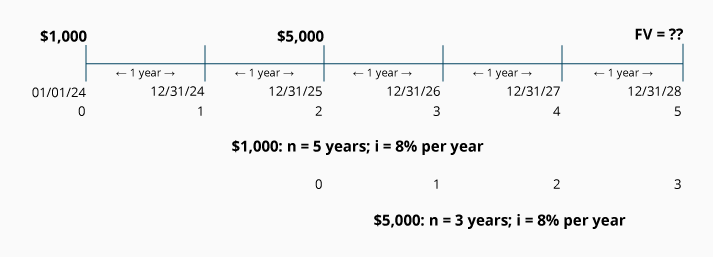The total future value on December 31, 2025 is the sum of these two calculations:

Future value calculation of the \$1,000 deposited on Jan 1, 2021: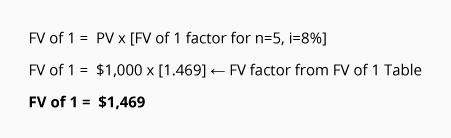Future value calculation of the \$5,000 deposited on Jan 1, 2023: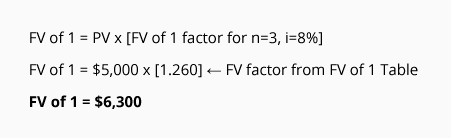The total future value on December 31, 2025 for these two deposits will be \$7,769. You can verify the future value of \$7,769 with the following table: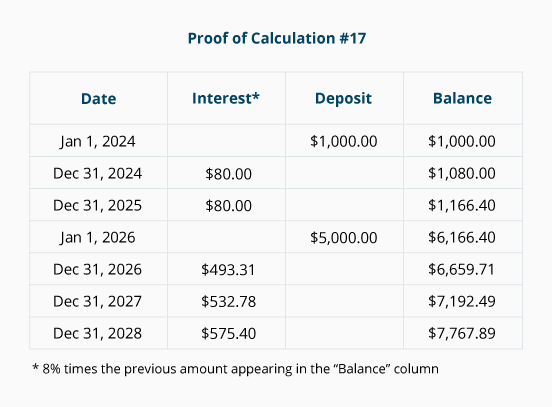### Calculation #18

You are asked to determine the total future value on December 31, 2025 of the \$1,000 deposit made on January 1, 2021 plus the \$5,000 deposit made on December 31, 2022. Both amounts will earn 8% per year compounded quarterly.

Because the interest is compounded quarterly, we convert the first deposit from 5 years to 20 quarterly periods, and the second deposit from 3 years to 12 quarterly periods. We convert the interest rate of 8% per year to the rate of 2% per quarter.

The following calculations reflect the restatement to quarters. Again, the sum of the answers to these two equations will be the future value on December 31, 2025.

Future value calculation of the \$1,000 deposited on Jan 1, 2021: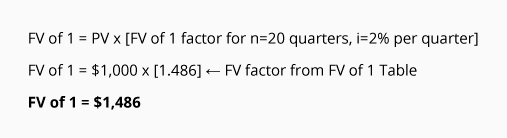Future value calculation of the \$5,000 deposited on Dec 31, 2022: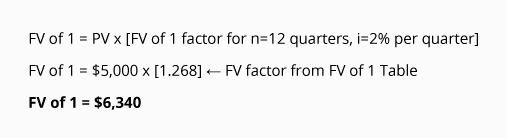The total future value on December 31, 2025 for these two deposits will be \$7,826. You can verify the future value of \$7,826 with the following table: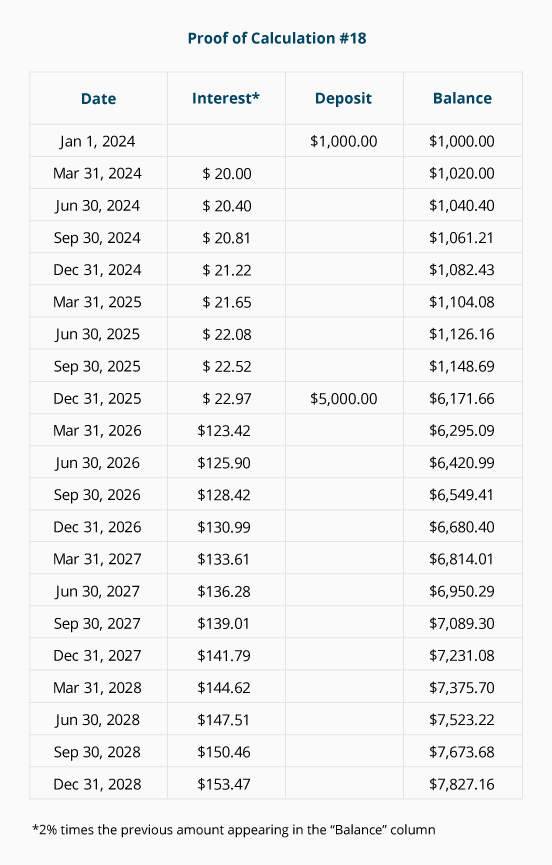#### Take Our Practice Quiz

We recommend that you now take our free Practice Quiz for this topic so that you can...

• See what you know
• See what you don't know